# Uncertainty, Error and Confidence in Data

Jim Sturgiss provides a straightforward guide to teaching some scientific concepts that are now part of the new Science syllabuses…

Uncertainty is a statistical concept found in the Assessing data and information outcome of the new Science syllabuses:.

WS 5.2 assess error, uncertainty and limitations in data (ACSBL004, ACSBL005, ACSBL033, ACSBL099)
This paper addresses uncertainty as a means of describing the accuracy of a series of measurements or as a means of comparing two sets of data. Uncertainty, or confidence, is described in terms of mean and standard deviation of a dataset. Standard deviation is a concept encountered by students in Stage 5.3 Mathematics and Stage 6 Standard 2 Mathematics.
Not explored in this paper is the use of Microsoft Excel or Google Sheets which can calculate uncertainty of datasets with ease (=STDEV.S(number1, number2,…).

Figure 1 Karl PearsonKarl Pearson (Figure 1), the great 19th-century biostatistician and eugenist, first described mathematical methods for determining the probability distributions of scientific measurements, and these methods form the basis of statistical applications in scientific research. Statistical techniques allow us to estimate uncertainty and report the error surrounding a value after repeated measurement of that value.

## 1. Accuracy, Precision and Error

Accuracy is how close a measurement is to the correct value for that measurement. The precision of a measurement system refers to how close the agreement is between repeated measurements (which are repeated under the same conditions). Measurements can be both accurate and precise, accurate but not precise, precise but not accurate, or neither.

### Precision and Imprecision

Precision (see Figure 2) refers to how well measurements agree with each other in multiple tests. Random error, or Imprecision, is usually quantified by calculating the coefficient of variation from the results of a set of duplicate measurements.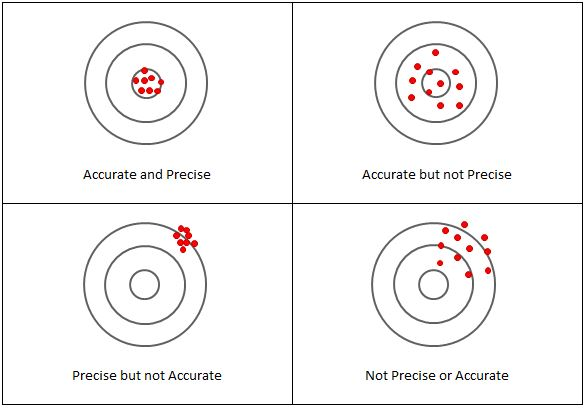Figure 2 Accuracy and precision

The accuracy of a measurement is how close a result comes to the true value.

### Error

When randomness is attributed to errors, they are “errors” in the sense in which that term is used in statistics.

• Systematic error (bias) occurs with the same value, when we use the instrument in the same way (eg calibration error) and in the same case. This is sometimes called statistical bias.

It may often be reduced with standardized procedures. Part of the learning process in the various sciences is learning how to use standard instruments and protocols so as to minimize systematic error.

• Random error, which may vary from one observation to another. Random error (or random variation) is due to factors which cannot, or will not, be controlled. Random error often occurs when instruments are pushed to the extremes of their operating limits. For example, it is common for digital balances to exhibit random error in their least significant digit. Three measurements of a single object might read something like 0.9111g, 0.9110g, and 0.9112g.

Systematic error or Inaccuracy (see Figure 3) is quantified by the average difference (bias) between a set of measurements obtained with the test method with a reference value or values obtained with a reference method.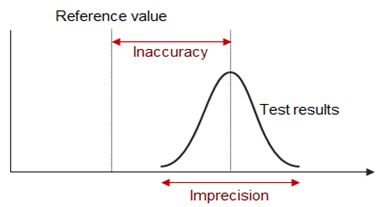Figure 3 Imprecision and in accuracy

## 2. Uncertainty

There is uncertainty in all scientific data. Uncertainty is reported in terms of confidence.

• Uncertainty is the quantitative estimation of error present in data; all measurements contain some uncertainty generated through systematic error and/or random error.
• Acknowledging the uncertainty of data is an important component of reporting the results of scientific investigation.
• Careful methodology can reduce uncertainty by correcting for systematic error and minimizing random error. However, uncertainty can never be reduced to zero.

### Estimating the Experimental Uncertainty For a Single Measurement

Any measurement made will have some uncertainty associated with it, no matter the precision of the measuring tool. So how is this uncertainty determined and reported?

The uncertainty of a single measurement is limited by the precision and accuracy of the measuring instrument, along with any other factors that might affect the ability of the experimenter to make the measurement.

For example, if you are trying to use a ruler to measure the diameter of a tennis ball, the uncertainty might be ± 5 mm, but if you used a Vernier caliper, the uncertainty could be reduced to maybe ± 2 mm. The limiting factor with the ruler is parallax, while the second case is limited by ambiguity in the definition of the tennis ball’s diameter (it’s fuzzy!). In both of these cases, the uncertainty is greater than the smallest divisions marked on the measuring tool (likely 1 mm and 0.05 mm respectively).

Unfortunately, there is no general rule for determining the uncertainty in all measurements. The experimenter is the one who can best evaluate and quantify the uncertainty of a measurement based on all the possible factors that affect the result. Therefore, the person making the measurement has the obligation to make the best judgment possible and to report the uncertainty in a way that clearly explains what the uncertainty represents:

Measurement = (measured value ± standard uncertainty) unit of measurement.
For example, where the ± standard uncertainty indicates approximately a 68% confidence interval, the diameter of the tennis ball may be written as 6.7 ± 0.2 cm.
Alternatively, where the ± standard uncertainty indicates approximately a 95% confidence interval, the diameter of the tennis ball may be written as 6.7 ± 0.4 cm.

## Estimating the Experimental Uncertainty For a Repeated Measure (Standard Deviation).

Suppose you time the period of oscillation of a pendulum using a digital instrument (that you assume is measuring accurately) and find: T = 0.44 seconds. This single measurement of the period suggests a precision of ±0.005 s, but this instrument precision may not give a complete sense of the uncertainty. If you repeat the measurement several times and examine the variation among the measured values, you can get a better idea of the uncertainty in the period.

For example, here are the results of 5 measurements, in seconds: 0.46, 0.44, 0.45, 0.44, 0.41.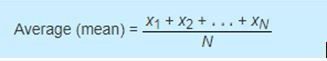For this situation, the best estimate of the period is the average, or mean.

Whenever possible, repeat a measurement several times and average the results. This average is generally the best estimate of the “true” value (unless the data set is skewed by one or more outliers). These outliers should be examined to determine if they are bad data points, which should be omitted from the average, or valid measurements that require further investigation.

Generally, the more repetitions you make of a measurement, the better this estimate will be, but be careful to avoid wasting time taking more measurements than is necessary for the precision required.

Consider, as another example, the measurement of the thickness of a piece of paper using a micrometer. The thickness of the paper is measured at a number of points on the sheet, and the values obtained are entered in a data table.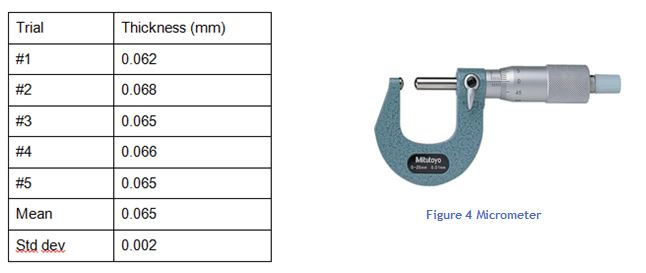This average is the best available estimate of the thickness of the piece of paper, but it is certainly not exact. We would have to average an infinite number of measurements to approach the true mean value, and even then, we are not guaranteed that the mean value is accurate because there is still some systematic error from the measuring tool, which can never be calibrated perfectly. So how do we express the uncertainty in our average value?

The most common way to describe the spread or uncertainty of the data is the standard deviation

Figure 5 Standard deviations of a normal distribution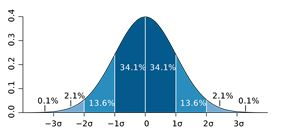The significance of the standard deviation is this:

if you now make one more measurement using the same micrometer, you can reasonably expect (with about 68% confidence) that the new measurement will be within 0.002 mm of the estimated average of 0.065 mm. In fact, it is reasonable to use the standard deviation as the uncertainty associated with this single new measurement.

This is written:
The thickness of 80 gsm paper (n=5) averaged 0.065 (s = 0.002mm)
s = standard deviation
OR
The thickness of 80 gsm paper (n=5) averaged 0.065 ± 0.004 mm to a 95% confidence level.
(0.004 mm represents 2 standard deviations, 2s)

## Standard Deviation of the Means (Standard Error of Mean (SEM))

The standard error is a measure of the accuracy of the estimate of the mean from the true or reference value. The main use of the standard error of the mean is to give confidence intervals around the estimated means for normally distributed data, not for the data itself but for the mean.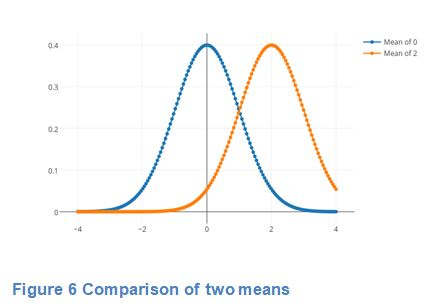If measured values are averaged, then the mean measurement value has a much smaller uncertainty, equal to the standard error of the mean, which is the standard deviation divided by the square root of the number of measurements.

Standard error is often us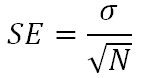ed to test (in terms of null hypothesis testing) differences between means.

For example, two populations of salmon fed on two different diets may be considered significantly different if the 95% confidence intervals (two std errors) around the estimated fish sizes under Diet A do not cross the estimated mean fish size under Diet B.Note that the standard error of the mean depends on the sample size, as the standard error of the mean shrinks to 0 as sample size increases to infinity.

Figure 7 Salmon

### Standard Error of Mean (SEM) Versus Standard Deviation

In scientific and technical literature, experimental data are often summarized either using the mean and standard deviation of the sample data or the mean with the standard error. This often leads to confusion about their interchangeability. However, the mean and standard deviation are descriptive statistics, whereas the standard error of the mean is descriptive of the random sampling process.

The standard deviation of the sample data is a description of the variation in measurements, whereas, the standard error of the mean is a probabilistic statement about how the sample size will provide a better bound on estimates of the population mean, in light of the central limit theorem.

Put simply, the standard error of the sample mean is an estimate of how far the sample mean is likely to be from the population mean, whereas the standard deviation of the sample is the degree to which individuals within the sample differ from the sample mean. If the population standard deviation is finite, the standard error of the mean of the sample will tend to zero with increasing sample size. This is because the estimate of the population mean will improve, while the standard deviation of the sample will tend to approximate the population standard deviation as the sample size increases.

### Confidence Levels

The confidence level represents the frequency (i.e. the proportion) of possible confidence intervals that contain the true value of the unknown population parameter. Most commonly, the 95.4% (“two sigma”) confidence level is used. However, other confidence levels can be used, for example, 68.3% (“one sigma”) and 99.7% (“three sigma”).

### Conclusion

Knowledge of normally distributed data and standard deviation are key to understanding the notions of statistical uncercertainty and confidence. These concepts are extended to the standard error of mean so that the significance of differences between two related datasets can be determined.

### Glossary

Absolute error The absolute error of a measurement is half of the smallest unit on the measuring device. The smallest unit is called the precision of the device.

Array An array is an ordered collection of objects or numbers arranged in rows and columns.

Bias This generally refers to a systematic favouring of certain outcomes more than others, due to unfair influence (knowingly or otherwise).

Confidence level The probability that the value of a parameter falls within a specified range of values. For example 2s = 95% confidence level.

Data cleansing Detecting and removing errors and inconsistencies from data in order to improve the quality of data (also known as data scrubbing).

Data set An organised collection of data.

Descriptive statistics These are statistics that quantitatively describe or summarise features of a collection of information.

Large data sets Data sets that must be of a size to be statistically reliable and require computational analysis to reveal patterns, trends and associations.

Limits of accuracy The limits of accuracy for a recorded measurement are the possible upper and lower bounds for the actual measurement.

Measures of central tendency Measures of central tendency are the values about which the set of data values for a particular variable are scattered. They are a measure of the centre or location of the data. The two most common measures of central tendency are the mean and the median.

Measures of spread Measures of spread describe how similar or varied the set of data values are for a particular variable. Common measures of spread include the range, combinations of quantiles (deciles, quartiles, percentiles), the interquartile range, variance and standard deviation.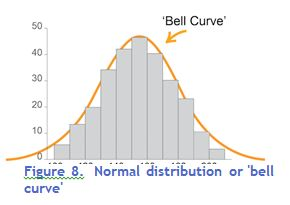Normal distribution The normal distribution is a type of continuous distribution whose graph looks like this:

The mean, median and mode are equal and the scores are symmetrically arranged either side of the mean.

The graph of a normal distribution is often called a ‘bell curve’ due to its shape.

Reliability An extent to which repeated observations and/or measurements taken under identical circumstances will yield similar results.

Sampling This is the selection of a subset of data from a statistical population. Methods of sampling include:

• systematic sampling – sample data is selected from a random starting point, using a fixed periodic interval
• self-selecting sampling – non-probability sampling where individuals volunteer themselves to be part of a sample
• simple random sampling – sample data is chosen at random; each member has an equal probability of being chosen
• stratified sampling – after dividing the population into separate groups or strata, a random sample is then taken from each group/strata in an equivalent proportion to the size of that group/strata in the population
• A sample can be used to estimate the characteristics of the statistical population.

Standard deviation This is a measure of the spread of a data set. It gives an indication of how far, on average, individual data values are spread from the mean.

Standard error The standard error of the mean (SEM) is the standard deviation of the sampling distribution of the mean.

Uncertainty Any single value has an uncertainty equal to the standard deviation. However, if the

values are averaged, then the mean measurement value has a much smaller uncertainty, equal to the standard error of the mean, which is the standard deviation divided by the square root of the number of measurements.

## Works Cited

Measurements and Error Analysis, www.webassign.net/question_assets/unccolphysmechl1/measurements/manual.html.

Altman, Douglas G, and J Martin Bland. “Standard Deviations and Standard Errors.” BMJ (Clinical Research Ed.), BMJ Publishing Group Ltd., 15 Oct. 2005, www.ncbi.nlm.nih.gov/pmc/articles/PMC1255808/.

Hertzog, Lionel. “Standard Deviation vs Standard Error.” DataScience , 28 Apr. 2017, https://datascienceplus.com/standard-deviation-vs-standard-error/

Mott, Vallerie. “Introduction to Chemistry.”
https://courses.lumenlearning.com/introchem/chapter/accuracy-precision-and-error/

Schoonjans, Frank. “Definition of Accuracy and Precision.” MedCalc, MedCalc Software, 9 Nov. 2018, www.medcalc.org/manual/accuracy_precision.php.

“Standard Error.” Wikipedia, Wikimedia Foundation, 7 Mar. 2019,
https://en.wikipedia.org/wiki/Standard_error

2336 | NSW Education Standards
https://educationstandards.nsw.edu.au/wps/portal/nesa/11-12/stage-6-learning-areas/stage-6-science/biology-2017/content/2336

Jim is an educational researcher and independent educational consultant. His M.Ed (Hons) thesis used an experimental design to evaluate the effectiveness of a literacy and learning program (1997). A recipient of the NSW Professional Teaching Council’s Distinguished Service Award for leadership in delivering targeted professional learning to teachers, he works with schools to align assessment, reporting and learning practice. He has been a head teacher of Science in two large Sydney high schools, as well as HSC Chemistry Senior Marker and Judge. For many years he served as a DoE Senior Assessment Advisor where he developed many statewide assessments, (ESSA, SNAP, ELLA, BST) and as Coordinator: Analytics where he developed reports to schools for statewide assessments and NAPLAN. He is a contributing author to the new Pearson Chemistry for NSW and to Macquarie University’s HSC Study Lab for Physics.